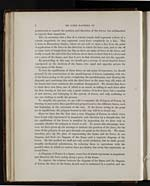# James Clerk Maxwell (1831-1879)

## On reciprocal figures, frames and diagrams of forces

2

MR CLERK MAXWELL ON

geometrical as regards the position and direction of the forces, but arithmetical
as regards their magnitude.

But, by assuming that a line of a certain length shall represent a force of a
certain magnitude, we may represent every force completely by a line. This
is done in Elementary Statics, where we are told to draw a line from the point
of application of the force in the direction in which the force acts, and to cut off
as many units of length from the line as there are units of force in the force, and
finally to mark the end of the line with an arrow-head, to show that it is a force and
not a piece of the frame, and that it acts in that direction and not the opposite.

By proceeding in this way, we should get a system of arrow-headed forces
superposed on the skeleton of the frame, two equal and opposite arrows for
every piece of the frame.

To test the equilibrium of these forces at any point of concourse, we should
proceed by the construction of the parallelogram of forces, beginning with two
of the forces acting at the point, completing the parallelogram, and drawing the
diagonal, and combining this with the third force in the same way, till, when all
the forces had been combined, the resultant disappeared. We should thus have
to draw three new lines, one of which is an arrow, in taking in each force after
the first, leaving at last not only a great number of useless lines, but a number
of new arrows, not belonging to the system of forces, and only confusing to
any one wishing to verify the process.

To simplify this process, we are told to construct the Polygon of Forces, by
drawing in succession lines parallel and proportional to the different forces, each
line beginning at the extremity of the last. If the forces acting at the point
are in equilibrium, the polygon formed in this way will be a closed one.

Here we have for the first time a true Diagram of Forces, in which every
force is not only represented in magnitude and direction by a straight line, but
the equilibrium of the forces is manifest by inspection, for we have only to
examine whether the polygon is closed or not. To secure this advantage, how-
ever, we have given up the attempt to indicate the position of the force, for the
sides of the polygon do not pass through one point as the forces do. We must,
therefore, give up the plan of representing the frame and its forces in one
diagram, and draw one diagram of the frame and a separate diagram of the
forces. By this method we shall not only avoid confusion, but we shall greatly
simplify mechanical calculations, by reducing them to operations with the
parallel ruler, in which no useless lines are drawn, but every line represents an
actual force.

A Diagram of Forces is a figure, every line of which represents in magnitude
and direction the force acting along a piece of the frame.

To express the relation between the diagram of the frame and the diagram
of forces, the lines of the frame should each be indicated by a symbol, and theView larger image

About this text